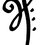# magical maths

Who doesn't love magic tricks? Here, we're going to look at how you can amaze your friends with some simple tricks involving numbers. You don't need a long-sleeve shirt, a rabbit, or even know the slightest bit of magic – just a little math.

Let's start off with a simple math trick.

Lightning Addition Proclaim to your friends: “I'm a human calculator! I can add five 3-digit numbers quicker than you can punch in the numbers in a calculator.” Then ask three friends to write down three separate and random 3-digit numbers. For instance:

240 520 842

Ask them to return the paper, upon which you add in the final two 3-digit numbers.

240 526 842 759 473

Race against your friends, who are armed with a calculator, to add all these numbers up, while you blurt out the answer within a matter of seconds: 2,840.

The secret: The last two numbers you throw in isn't random at all – it may look random to your friends, but you've carefully chosen them so that the fourth and first numbers add up to 999 (in the example above, it would be 759, since 240 + 759 = 999). Choose the fifth number so that it adds with the second number to give 999 (473 + 526 = 999).

Once you have that down, the rest is simple: the sum is 2,000, add the number in the middle, and subtract 2. And voila! 2,840.

Lightning Addition 2 If your friends weren't too impressed by that trick, try this one. Ask a friend to pick two different single-digit numbers (for example, 4 and 7) and add them up (4 + 7 = 11). Tell them to do it in secret, so that you can't see what numbers they chose, or what they're doing.

Now, ask them to take the sum of the first two numbers (11), and add it to the number prior to that (which is 7). So the chain of calculations now appears to be as such:

4 +7
+11 18

Continue on the chain of addition until he reaches 10 numbers.

4 +7
+11
+18
+29
+47
+76
+123 +199 +322 ????

Once done, ask your friend to show you the chain of numbers. Here comes the amazing part: challenge your friend, who's armed with a calculator, to figure the sum of all these 10 numbers, while you figure out the answer in less than five seconds – which is 836.

The secret: It turns out that this pattern of addition follows the Fibonacci sequence of numbers, which is the basis for the Fibonacci ratio. You might know it as the Golden Ratio, which often occurs in nature.

The trick here is that the seventh number in the sequence, multiplied by 11, will always equal the sum of the 10-number sequence. In this case, it's 76 x 11 = 836.

Wait a minute, you say. How does one multiply the number by 11 in under five seconds? Here's where you use another arithmetic trick: Take the two numbers, 7 and 6, and split it, leaving a blank space in the middle:

7 __ 6

In the space between, place the sum of the two numbers:

7 13 6

Because the space in between 7 and 6 can only have one digit, carry forward 1 to 7, and what you end up with is:

836

Simple? Try it with 48 x 11

4 __ 8

Place the sum of the two numbers

4 12 8

Then, carry forward the 1 to the 4

528

Note: If your friend starts off with 9 and 7, or 9 and 8, the seventh number would be a 3-digit number (101 and 109, respectively). In these special cases, just remember that the result after multiplying them by 11 is 1,111, and 1,199 respectivelyNote by Vishwathiga Jayasankar
6 years, 7 months ago

This discussion board is a place to discuss our Daily Challenges and the math and science related to those challenges. Explanations are more than just a solution — they should explain the steps and thinking strategies that you used to obtain the solution. Comments should further the discussion of math and science.

When posting on Brilliant:

• Use the emojis to react to an explanation, whether you're congratulating a job well done , or just really confused .
• Ask specific questions about the challenge or the steps in somebody's explanation. Well-posed questions can add a lot to the discussion, but posting "I don't understand!" doesn't help anyone.
• Try to contribute something new to the discussion, whether it is an extension, generalization or other idea related to the challenge.
• Stay on topic — we're all here to learn more about math and science, not to hear about your favorite get-rich-quick scheme or current world events.

MarkdownAppears as
*italics* or _italics_ italics
**bold** or __bold__ bold
- bulleted- list
• bulleted
• list
1. numbered2. list
1. numbered
2. list
Note: you must add a full line of space before and after lists for them to show up correctly
paragraph 1paragraph 2

paragraph 1

paragraph 2

[example link](https://brilliant.org)example link
> This is a quote
This is a quote
    # I indented these lines
# 4 spaces, and now they show
# up as a code block.

print "hello world"
# I indented these lines
# 4 spaces, and now they show
# up as a code block.

print "hello world"
MathAppears as
Remember to wrap math in $$ ... $$ or $ ... $ to ensure proper formatting.
2 \times 3 $2 \times 3$
2^{34} $2^{34}$
a_{i-1} $a_{i-1}$
\frac{2}{3} $\frac{2}{3}$
\sqrt{2} $\sqrt{2}$
\sum_{i=1}^3 $\sum_{i=1}^3$
\sin \theta $\sin \theta$
\boxed{123} $\boxed{123}$

## Comments

Sort by:

Top Newest

cant understand anything

- 6 years, 7 months ago

Log in to reply

×

Problem Loading...

Note Loading...

Set Loading...